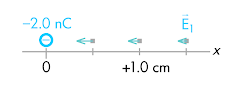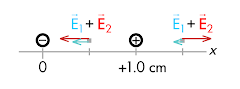## 20140313

### Physics quiz question: comparing total electric field magnitudes at separate locations

Physics 205B Quiz 3, spring semester 2014
Cuesta College, San Luis Obispo, CA

Cf. Giambattista/Richardson/Richardson, Physics, 2/e, Problem 16.33Two point charges are held at fixed locations. A –2.0 nC charge is at the origin, and a second +8.0 nC charge is at x = +1.0 cm. The magnitude of the electric field at x = +0.5 cm is __________ the magnitude of the electric field at x = +1.5 cm.
(A) less than.
(B) equal to.
(C) greater than.
(D) (Not enough information is given.)

Correct answer (highlight to unhide): (C)The electric field vectors due to the –2.0 nC source charge are shown at right, where they all point in towards the negative source charge. (The magnitudes of these electric field vectors are not quite to scale, but do get smaller with increasing distance from the –2.0 nC source charge).The electric field vectors due to the +8.0 nC source charge are shown at right, where they all point out away from the positive source charge. (The magnitudes of these electric field vectors are not quite to scale, but do get smaller with increasing distance from the +8.0 nC source charge, and are proportionally larger than the electric field vectors of the less –2.0 nC source charge).The total electric field at x = +0.5 cm and x = +1.5 cm due to both source charges is the vector addition of the individual electric field vectors at each location. Note that the magnitudes of the electric field vectors from the +8.0 nC source charge are the same at both x = +0.5 cm and x = +1.5 cm (as these locations are the same distance from the +8.0 nC source charge).

Note that the magnitude of the total electric field at x = +0.5 cm would be greater than the magnitude of the electric field due to the +8.0 nC source charge, as the electric field due to the –2.0 nC source charge points in the same direction (to the left), such that their magnitudes would add together.

In contrast, the total electric field at x = +1.5 cm would be less than the magnitude of the electric field due to the +8.0 nC source charge, as the electric field due to the –2.0 nC source charge points in the opposite direction (to the left), such that its magnitude would subtract from the magnitude of the electric field due to the +8.0 nC source charge.

Sections 30882, 30883
Exam code: quiz03cDvD